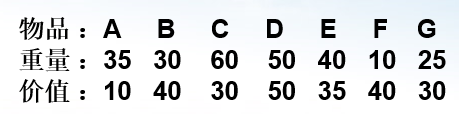## 二周总结—贪心算法+浅谈动态规划

#贪心算法（一种特殊的动态规划，由于其具有贪心选择性质，保证了子问题只会被计算一次，不会被多次计算，因此贪心算法其实是最简单的动态规划。）

1.建立数学模型来描述题目（从所得模型中找出可以贪的地方）；
2.把求解的问题分为若干子问题（从局部最优入手）；
3.对每一子问题的最优求解（贪的实现）；
4.把子问题的解局部最优解合成原来解问题的一个解（但不一定使最终结果最优）；

1.有一个背包，背包容量是M=150。有7个物品，物品可以分割成任意大小。要求尽可能让装入背包中的物品总价值最大，但不能超过总容量。``````#include <iostream>
#include <algorithm>
#include <stdio.h>
using namespace std;
struct kd      //结构体来描述物品的信息，n为重量，v为价，x为性价比也是我们贪的比较；
{
int n;
int v;
double x;
}a;
bool cap(kd a,kd b)    //比较函数，为下面的排序函数定规则；
{
if(a.x!=b.x) return a.x>b.x;
else return a.n>b.n;
}
int main()
{
int k,w,s,i,j;    //k为测试组数，w为背包空间，s为物品件数
cin>>k;
double b[k]={0};  //用来储存最后的价值总额；
for(i=0;i<k;i++)
{
cin>>w>>s;
for(j=0;j<s;j++)
{
cin>>a[j].n>>a[j].v;
a[j].x=1.0*a[j].v/a[j].n;
}
sort(a,a+s,cap);    //对输入的物品进行性价比从高到低的排序；
for(j=0;j<s;j++)
{
if(a[j].n>w)
{
b[i]+=w*a[j].x;
break;
}
else
{
b[i]+=a[j].n*a[j].x;
w-=a[j].n;
}
}
}
for(i=0;i<k;i++)
printf("%.2f\n", b[i]);
return 0;
}``````

2.均分纸牌：有N堆纸牌，编号分别为1，2，…，n。每堆上有若干张,但纸牌总数必为n的倍数.可以在任一堆上取若干张纸牌,然后移动。移牌的规则为：在编号为1上取的纸牌，只能移到编号为2的堆上；在编号为n的堆上取的纸牌，只能移到编号为n-1的堆上；其他堆上取的纸牌，可以移到相邻左边或右边的堆上。现在要求找出一种移动方法，用最少的移动次数使每堆上纸牌数都一样多。例如：n=4，4堆纸牌分别为：① 9 ② 8 ③ 17 ④ 6 移动三次可以达到目的：从③取4张牌放到④ 再从③区3张放到②然后从②去1张放到①。

1．若a[i]>v，则将a[i]-v张从第I堆移动到第I+1堆；

2．若a[i]<v，则将v-a[i]张从第I+1堆移动到第I堆。

``````#include <iostream>
using namespace std;
int main()
{
int n,i,a,p,s=0,b=0;    //n为堆数，a[i]存每堆的牌数，s为总牌数，p为每堆的平均牌数，b为所需步数；
cin>>n;
for(i=0;i<n;i++)
{
cin>>a[i];
s+=a[i];
}
p=s/n;
for(i=0;i<n;i++)
{
if(a[i]!=p)
{
a[i+1]+=a[i]-p; //有贪心思想的数学公式，也是每后一堆可以接受上一堆多的，也可以移给上一堆少的；
a[i]=p;         //移后真是所保留的平均牌数；
b++;
}
}
cout<<b<<endl;
return 0;
}``````

1.金银岛[题目]：(http://sdau.openjudge.cn/tx/03/)：与背包问题的解决方法一样，从性价比高的开始取。

2.[题目]：(http://sdau.openjudge.cn/tx/11/)：N头奶牛，每头奶牛有自己的高度Hi，N头奶牛的总高度为S。书架高度为B(1 ≤ B ≤ S < 2,000,000,007)用最少的牛叠出比N高。

``````#include <iostream>
#include <algorithm>
using namespace std;
int main()
{
int n,b,i,a,d=0,s=0;
cin>>n>>b;
for(i=0;i<n;i++)
cin>>a[i];
sort(a,a+n);             //从小到大排序，只需最后从大到小取出；
for(i=n-1;d<b;i--,s++)
d+=a[i];
cout<<s<<endl;
return 0;
}
``````

3.装箱问题：[题目]：(http://sdau.openjudge.cn/tx/05/)

``````#include <iostream>
#include <stdio.h>
#include <cmath>
using namespace std;
int p1,p2,p3,p4,p5,p6,s;  //定义7个全局变量好进行对不同组数据的验算；
int main()
{
while(scanf("%d%d%d%d%d%d",&p1,&p2,&p3,&p4,&p5,&p6)&&p1+p2+p3+p4+p5+p6) //对数据经行输入，同时判断结束的条件输入000000时；
{
s=0;
s+=p6+p5+p4;      //大箱子一次只能装一个，剩的空间用小的填补；
p1-=min(p1,p5*11);  //取两者之间小的哪一个，也是装咯p5的剩下用1*1的经行填补；
if(p2<p4*5)         //装咯4*4的剩下的填补情况；
p1-=min(p1,(p4*5-p2)*4);
p2-=min(p2,p4*5);
s+=ceil(p3/4.0);    //装3*3的个数；
p3%=4;
if(p3==1)           //填补装咯3*3的剩余空间的填补；
{
if(p2<5)
p1-=min(p1,(5-p2)*4);
p1-=min(p1,7);
p2-=min(p2,5);
}
if(p3==2)
{
if(p2<3)
p1-=min(p1,(3-p2)*4);
p1-=min(p1,6);
p2-=min(p2,3);
}
if(p3==3)
{
if(p2>=0)
p1-=min(p1,4);
p1-=min(p1,5);
p2-=min(p2,1);
}
s+=ceil(p2/9.0);    //对余下的2*2进行装箱；
p2%=9;
if(p2)
p1-=min(p1,(9-p2)*4);
s+=ceil(p1/36.0);  //余下的1*1进行装箱；
cout<<s<<endl;
}
}``````

4.电池寿命：[题目]：(http://sdau.openjudge.cn/tx/12/)

1）如果成立的话那当然就是剩下的所有电池与最大的电池车轮战，最大值为n-1个数的和，
2）如果不成立的话那么最大就是n个数的和的一半，也就是说电池电量是一定可以全部用完的。

``````#include <iostream>
#include <cmath>
#include <stdio.h>
using namespace std;
int main()
{
int n,x,maxn,s,s1;
while(cin>>n)
{
s=0;
maxn=0;
for(int i=0;i<n;i++)
{
cin>>x;
maxn=max(maxn,x);
s+=x;
}
s1=s-maxn;
if(s1<maxn)
printf("%.1f\n", (double)s1);
else printf("%.1f\n", s/2.0);
}
return 0;
}``````

##动态规划：动态规划问题是面试题中的热门话题，如果要求一个问题的最优解（通常是最大值或者最小值），而且该问题能够分解成若干个子问题，并且小问题之间也存在重叠的子问题，则考虑采用动态规划。

1）重叠子问题 1）贪心选择性质
2）最优子结构 2）最优子结构

1. 求一个问题的最优解 ；
2. 大问题可以分解为子问题，子问题还有重叠的更小的子问题 ；
3. 整体问题最优解取决于子问题的最优解（状态转移方程） ；
4. 从上往下分析问题，从下往上解决问题 ；
5. 讨论底层的边界问题；
动态规划需要注意的要点：
1）状态（小规模问题的数学表示）；
2）状态转移方程（大规模问题如何转化为更小的问题）；
3）最小状态（最小规模的问题）；
4）要求的返回值是什么；
1、动态规划的设计，其实就是利用最优子结构和重叠子问题性质对穷举法进行优化，通过将中间结果保存在数组中，实现用空间来换取时间交换，实现程序的快速运行。（动态规划求解时，一般都会转化为网格进行求解，而且因为是空间换时间（避免了子问题的重复计算），因此一般迭代求解）。2、一般对所要求解的问题的时间复杂度要求比较高的，可以优先考虑如下几种算法。①分治法②递归法③贪心算法④动态规划算法
动态规划杀手锏：1）建模：最优子结构状态转移方程边界2）实现：递归法备忘录法（从上倒下，非全二叉树，hash保存！）自底而上（迭代实现）